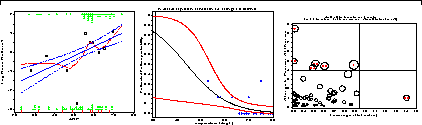# Chapter 6. Logistic regressionLogistic regression describes the relation between a discrete response, often binary, and a set of explanatory variables. Smoothing techniques are often crucial in visualizations for such discrete data. The fitted model provides both inference and prediction, accompanied by measures of uncertainty. Diagnostic plots help us to detect influential observations which may distort our results. 6.1. The logistic regression model 6.1.1. Plotting a discrete response: the LOGODDS macro 6.1.2. Plotting a discrete response: Easy smoothing with PROC GPLOT 6.2. Models for quantitative predictors 6.2.1. Fitting logistic regression models 6.2.2. Plotting predicted probabilities 6.3. Logit models for qualitative predictors 6.3.1. Plotting results from PROC LOGISTIC 6.4. Multiple logistic regression models 6.4.1. Models with interaction 6.4.2. Effect plots from coefficients 6.5. Influence and diagnostic plots 6.5.1. Residuals and leverage 6.5.2. Influence diagnostics 6.5.3. Influence output from PROC LOGISTIC 6.5.4. Diagnostic plots of influence measures 6.5.5. Partial residual and added-variable plots 6.6. Polytomous response models 6.6.1. Ordinal response 6.6.2. Plotting results from PROC LOGISTIC 6.6.3. Nested dichotomies 6.6.4. Generalized Logits 6.7. The Bradley-Terry-Luce Model for Paired Comparisons 6.8. Power and sample size for logistic regression 6.8.1. Binary predictor: Comparing two proportions 6.8.2. Quantitative predictor 6.9. Chapter summary